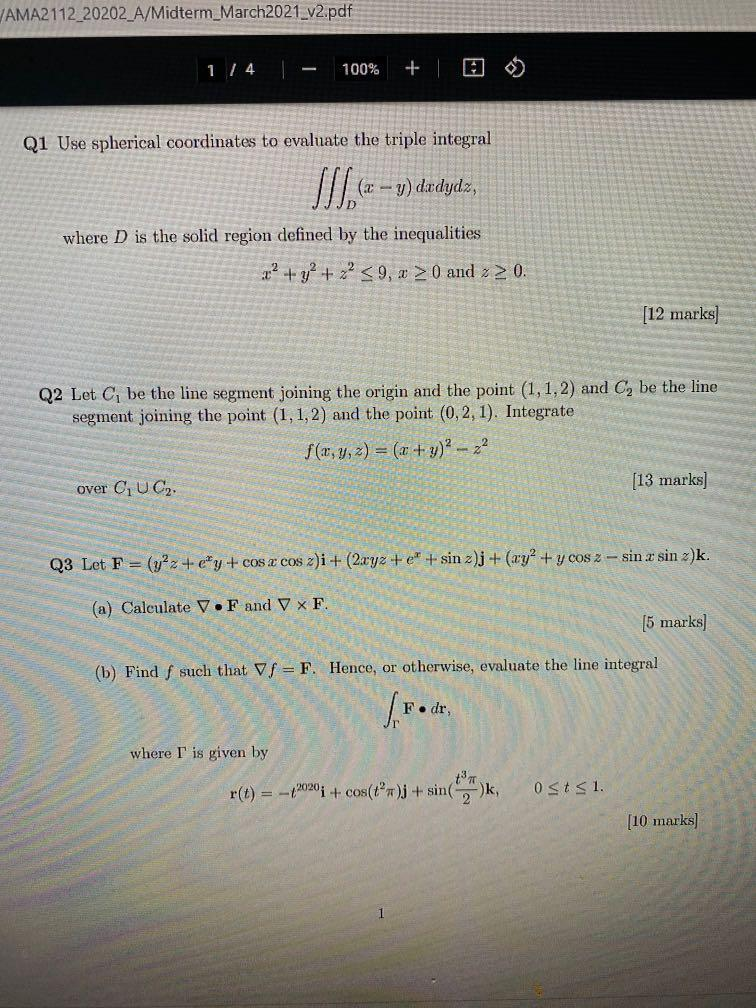# Question AMA2112_20202_A/Midterm_March2021_v2.pdf 1/4 100% + Q1 Use spherical coordinates to evaluate the triple integral - y) dxdydz, where D is the solid region defined by the inequalities 72 + y2 + x2 <9, x > 0 and 2 2 0. [12 marks] Q2 Let C, be the line segment joining the origin and the point (1,1,2) and C, be the line segment joining the point (1,1,2) and the point (0,2,1). Integrate f(x, y, z) = (x + y)2 - 22 over CUC2 [13 marks] Q3 Let F = (y2 + y + cos x cos z)i + (2.xyz + e + sin 2)j + (xy + y cos 2 - sin x sin 2)k. (a) Calculate V.F and V X F. [5 marks) (b) Find f such that f= F. Hence, or otherwise, evaluate the line integral where T is given by r(t) = –2020 + cos(tºn)j + sin(-)k, Ost 51. [10 marks]RXAXAW The Asker · Other MathematicsTranscribed Image Text: AMA2112_20202_A/Midterm_March2021_v2.pdf 1/4 100% + Q1 Use spherical coordinates to evaluate the triple integral - y) dxdydz, where D is the solid region defined by the inequalities 72 + y2 + x2 <9, x > 0 and 2 2 0. [12 marks] Q2 Let C, be the line segment joining the origin and the point (1,1,2) and C, be the line segment joining the point (1,1,2) and the point (0,2,1). Integrate f(x, y, z) = (x + y)2 - 22 over CUC2 [13 marks] Q3 Let F = (y2 + y + cos x cos z)i + (2.xyz + e + sin 2)j + (xy + y cos 2 - sin x sin 2)k. (a) Calculate V.F and V X F. [5 marks) (b) Find f such that f= F. Hence, or otherwise, evaluate the line integral where T is given by r(t) = –2020 + cos(tºn)j + sin(-)k, Ost 51. [10 marks]
More
Transcribed Image Text: AMA2112_20202_A/Midterm_March2021_v2.pdf 1/4 100% + Q1 Use spherical coordinates to evaluate the triple integral - y) dxdydz, where D is the solid region defined by the inequalities 72 + y2 + x2 <9, x > 0 and 2 2 0. [12 marks] Q2 Let C, be the line segment joining the origin and the point (1,1,2) and C, be the line segment joining the point (1,1,2) and the point (0,2,1). Integrate f(x, y, z) = (x + y)2 - 22 over CUC2 [13 marks] Q3 Let F = (y2 + y + cos x cos z)i + (2.xyz + e + sin 2)j + (xy + y cos 2 - sin x sin 2)k. (a) Calculate V.F and V X F. [5 marks) (b) Find f such that f= F. Hence, or otherwise, evaluate the line integral where T is given by r(t) = –2020 + cos(tºn)j + sin(-)k, Ost 51. [10 marks]